Next: Spectrum of a pole Up: DAMPED OSCILLATION Previous: Narrow-band filters

## Polynomial division

Convolution with the coefficients bt of B(Z)=1/A(Z) is a narrow-banded filtering operation. If the pole is chosen very close to the unit circle, the filter bandpass becomes very narrow, and the coefficients of B(Z) drop off very slowly. A method exists of narrow-band filtering that is much quicker than convolution with bt. This is polynomial division by A(Z). We have for the output Y(Z):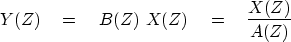(30)
Multiply both sides of (30) by A(Z):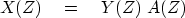(31)
For definiteness, let us suppose that the xt and yt vanish before t = 0. Now identify coefficients of successive powers of Z to get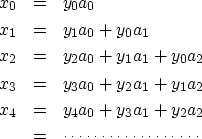(32)
Let Na be the highest power of Z in A(Z). The k-th equation (where k>Na) is(33)
Solving for yk, we get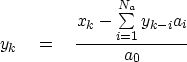(34)
Equation (34) may be used to solve for yk once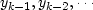are known. Thus the solution is recursive. The value of Na is only 2, whereas Nb is technically infinite and would in practice need to be approximated by a large value. So the feedback operation (34) is much quicker than convolving with the filter B(Z)=1/A(Z). A program for the task is given below. Data lengths such as na in the program polydiv() include coefficients of all Na powers of Z as well as 1=Z0, so na = Na+1.
#       polynomial division feedback filter:    Y(Z) = X(Z) / A(Z)
#
subroutine polydiv( na, aa, nx, xx, ny, yy )
integer na      # number of coefficients of denominator
integer nx      # length of the input function
integer ny      # length of the output function
real    aa(na)  # denominator recursive filter
real    xx(nx)  # input trace
real    yy(ny)  # output trace, as long as input trace.

integer ia, iy
do iy= 1, ny
if( iy <= nx)
yy(iy) = xx(iy)
else
yy(iy) = 0.
do iy= 1, na-1  {                                       # lead-in terms
do ia= 2, iy
yy(iy) = yy(iy) - aa(ia) * yy(iy-ia+1)
yy(iy) = yy(iy) / aa(1)
}
do iy= na, ny {                                         # steady state
do ia= 2, na
yy(iy) = yy(iy) - aa(ia) * yy(iy-ia+1)
yy(iy) = yy(iy) / aa(1)
}
return; endNext: Spectrum of a pole Up: DAMPED OSCILLATION Previous: Narrow-band filters
Stanford Exploration Project
10/21/1998